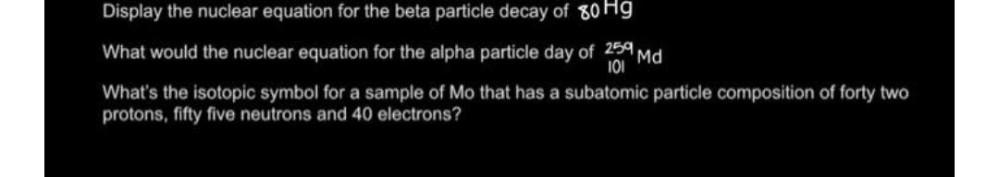Question:

# Display the nuclear equation for the beta particle decay of 80 Hg What would the nuclear equation for the alpha particle day ofDisplay the nuclear equation for the beta particle decay of 80 Hg What would the nuclear equation for the alpha particle day of 259 Md What's the isotopic symbol for a sample of Mo that has a subatomic particle composition of forty two protons, fifty five neutrons and 40 electrons ?